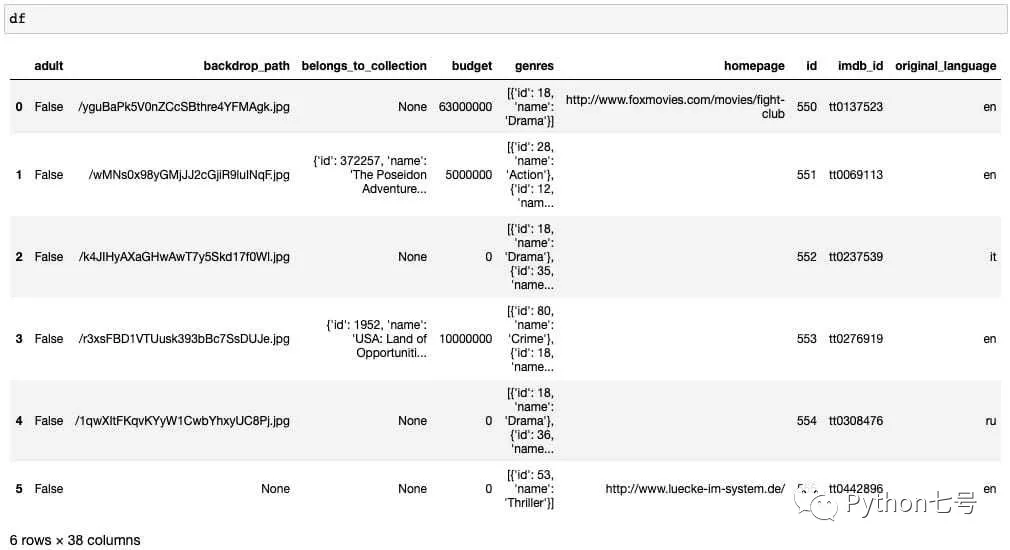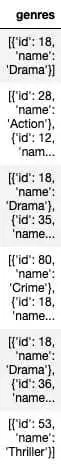# 用 Pandas 做 ETL，不要太快

ETL 的全称是 extract, transform, load，意思就是：提取、转换、 加载。ETL 是数据分析中的基础工作，获取非结构化或难以使用的数据，把它变为干净、结构化的数据，比如导出 csv 文件，为后续的分析提供数据基础。

1、提取数据

#config.py
api_key =

import pandas as pd
import requests
import config

API_KEY = config.api_key
url = 'https://api.themoviedb.org/3/movie/{}?api_key={}'.format(movie_id, API_KEY)

r = requests.get(url)

response_list = []
API_KEY = config.api_key

for movie_id in range(550,556):
url = 'https://api.themoviedb.org/3/movie/{}?api_key={}'.format(movie_id, API_KEY)
r = requests.get(url)
response_list.append(r.json())

df = pd.DataFrame.from_dict(response_list)2、转换

budget
id
imdb_id
genres
original_title
release_date
revenue
runtime

df_columns = ['budget', 'genres', 'id', 'imdb_id', 'original_title', 'release_date', 'revenue', 'runtime']genres_list = df['genres'].tolist()
flat_list = [item for sublist in genres_list for item in sublist]

result = []
for l in genres_list:
r = []
for d in l:
r.append(d['name'])
result.append(r)
df = df.assign(genres_all=result)

df_genres = pd.DataFrame.from_records(flat_list).drop_duplicates()df_columns = ['budget', 'id', 'imdb_id', 'original_title', 'release_date', 'revenue', 'runtime']
df_genre_columns = df_genres['name'].to_list()
df_columns.extend(df_genre_columns)

s = df['genres_all'].explode()
df = df.join(pd.crosstab(s.index, s))df['release_date'] = pd.to_datetime(df['release_date'])
df['day'] = df['release_date'].dt.day
df['month'] = df['release_date'].dt.month
df['year'] = df['release_date'].dt.year
df['day_of_week'] = df['release_date'].dt.day_name()
df_time_columns = ['id', 'release_date', 'day', 'month', 'year', 'day_of_week']3、加载

df[df_columns].to_csv('tmdb_movies.csv', index=False)
df_genres.to_csv('tmdb_genres.csv', index=False)
df[df_time_columns].to_csv('tmdb_datetimes.csv', index=False)

Pandas 是处理 excel 或者数据分析的利器，ETL 必备工具，本文以电影数据为例，分享了 Pandas 的常见用法。

## 官方微博/微信↑扫描二维码

1.用手机扫左侧二维码；

2.在添加朋友里，搜索关注TechWeb。